# How to fix scipys interpolating spline default behavior

Scipy’s UnivariateSpline class is a super useful way to smooth time series, especially if you need an estimate of the derivative. It is an implementation of an interpolating spline, which I’ve previously covered in this blog post. Its big problem is that the default parameters suck. Depending on the absolute value of your data, the spline produced by leaving the parameters at their default values can be overfit, underfit or just fine. Below I visually reproduce the problem for two time series from an experiment with very different numerical values.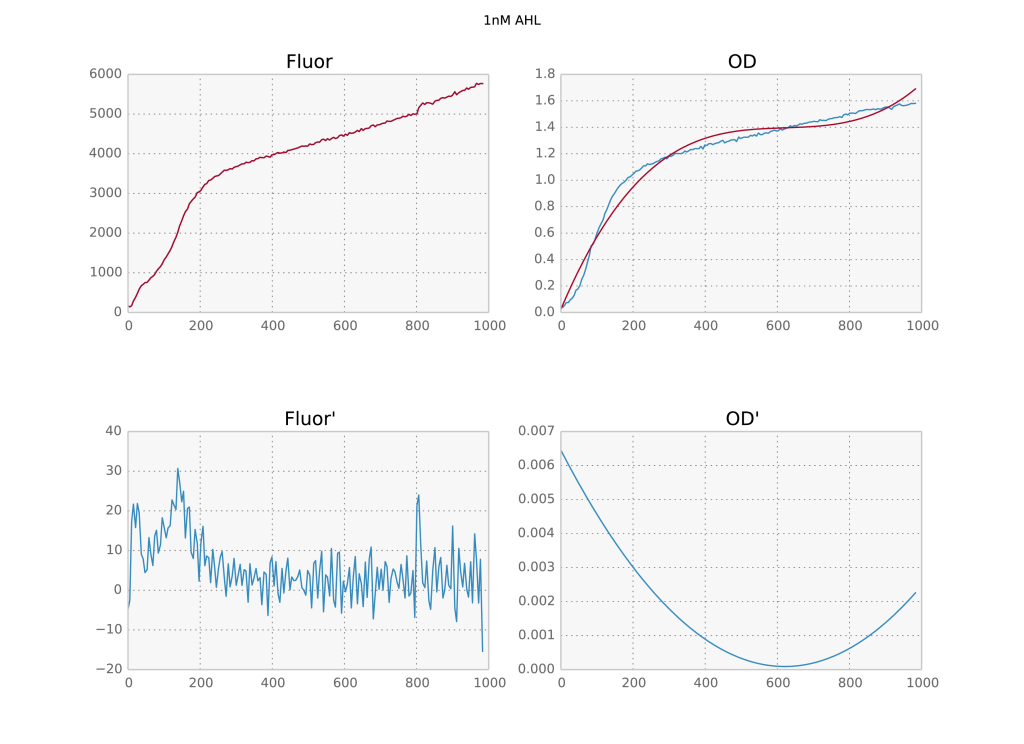Two time series with different numerical values and their derivatives below. The first is overfit, the second underfit.

My usual solution was just to manually adjust the $s$ parameter until the result looked good. But this time I have hundreds of time series, so I have to do it right this time. And doing it right requires actually understanding what’s going on. In the documentation, $s$ is described as follows:

Positive smoothing factor used to choose the number of knots. Number of knots will be increased until the smoothing condition is satisfied:

sum((w[i]*(y[i]-s(x[i])))**2,axis=0) <= s

If None (default), s=len(w) which should be a good value if 1/w[i] is an estimate of the standard deviation of y[i]. If 0, spline will interpolate through all data points.

So the default value of $s$ should be fine if $w^{-1}$ were an estimate of the standard deviation of $y$. However, the default value for $w$ is 1/len(y) which is clearly not a decent estimate. The solution then is to calculate a rough estimate of the standard deviation of $y$ and pass the inverse of that as $w$. My solution to that is to use a gaussian kernel to smooth the data and then calculate a smoothed variance as well. Code below:

def moving_average(self, series, sigma=3):
b = gaussian(39, sigma)
average = filters.convolve1d(series, b/b.sum())
var = filters.convolve1d(np.power(series-average,2), b/b.sum())
return average, var

_, var = moving_average(series)
sp = ip.UnivariateSpline(x, series, w=1/np.sqrt(var))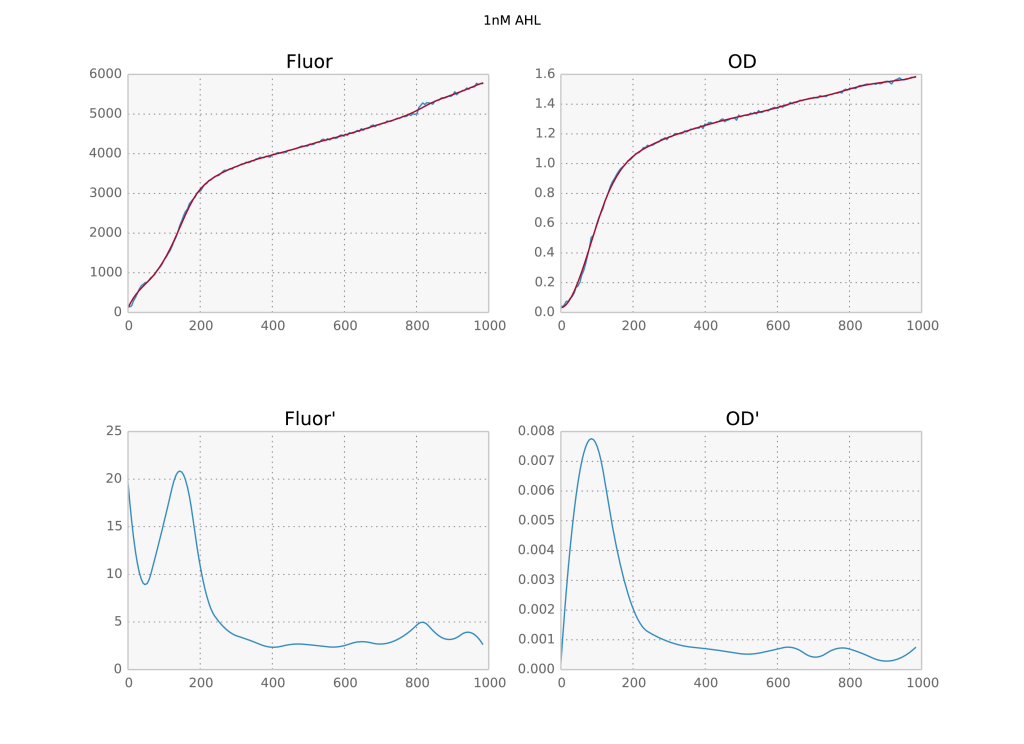Now, you may be thinking I only moved the parameter dependence around: before I had to fine tune $s$ but now there is a free parameter sigma. The difference is that a) the gaussian filter results are much more robust with respect to the choice of sigma;  b) we only need to provide an estimate of the standard deviation, so it’s fine if the result coming out is not perfect; and c) it does not depend on the absolute value of the data. In fact, for the above dataset I left sigma at its default value of 3 for all timeseries and all of them came out perfect. So I’d consider this problem solved.

I understand why the scipy developers wouldn’t use a method similar to mine to estimate $w$ as default, after all it may not work for all types of data. On the other hand, I think the documentation as it stands is confusing. The user would expect that parameters which have a default value would work without fine tuning, instead what happens here is that if you leave $w$ as the default you must change $s$ and vice versa.

# Quick introduction to gaussian mixture models with python

Usually we like to model probability distributions with gaussian distributions. Not only are they the maximum entropy distributions if we only know the mean and variance of a dataset, the central limit theorem guarantees that random variables which are the result of summing many different random variables will be gaussian distributed too. But what to do when we have multimodal distributions like this one?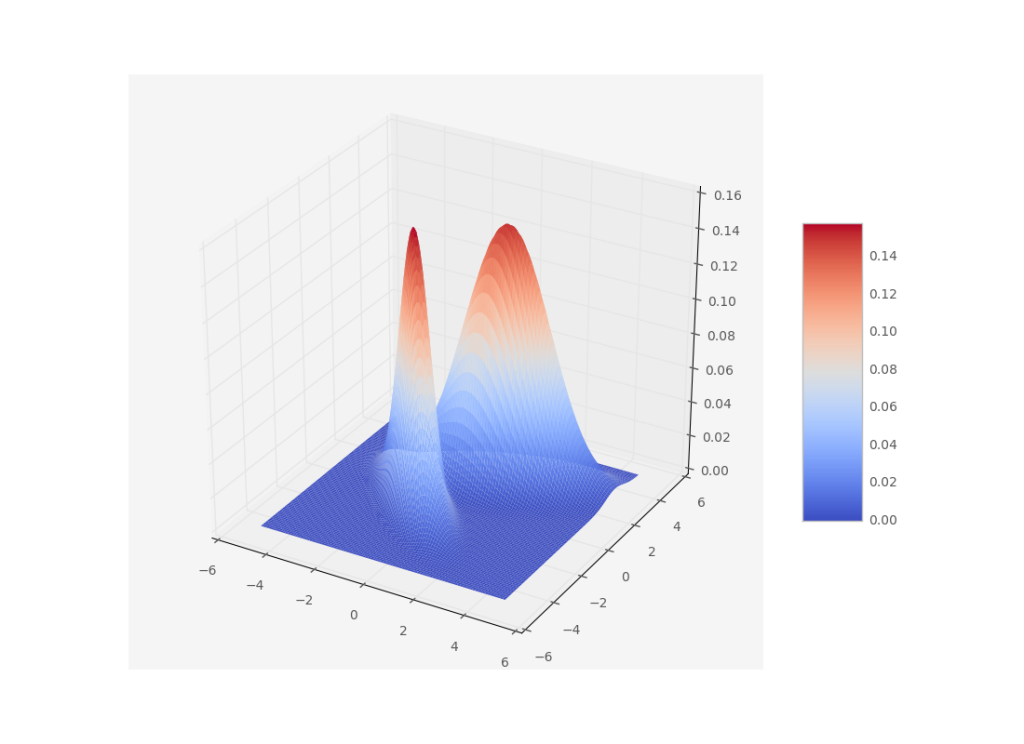A gaussian distribution would not represent this very well. So what’s the next best thing? Add another gaussian! A gaussian mixture model is defined by a sum of gaussians

$$P(x)=\sum_i w_i \, \mathcal{G}(\mu_i, \Sigma_i)$$

with means $\mu$ and covariance matrices $\Sigma$.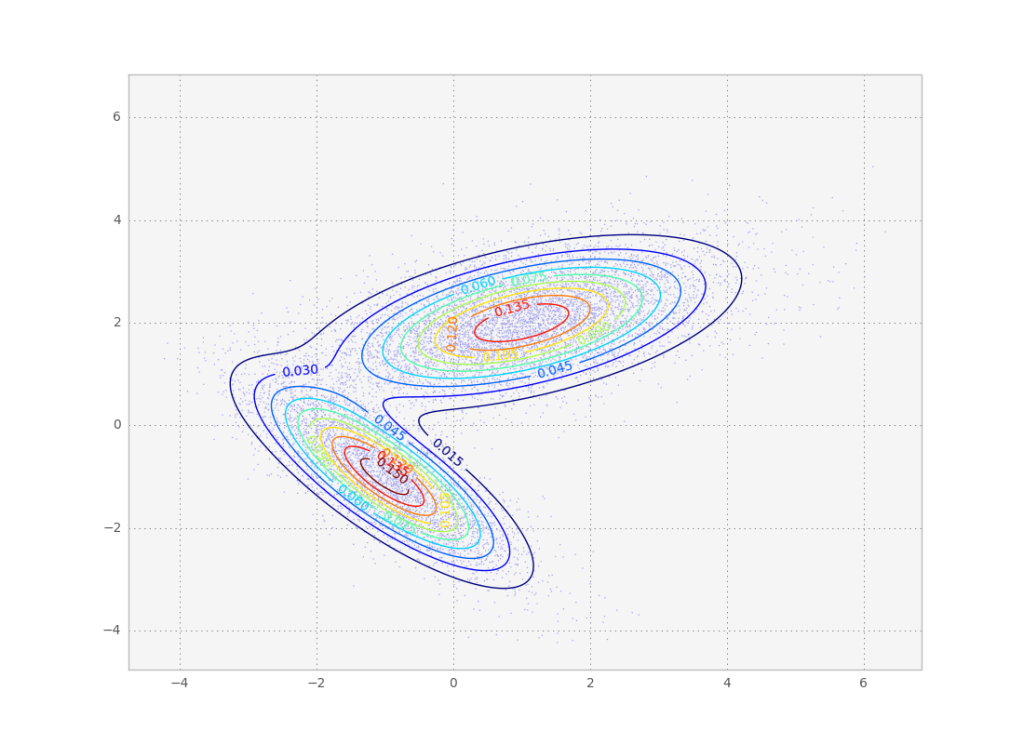The above gaussian mixture can be represented as a contour plot. Note this is the same distribution we sampled from in the metropolis tutorial.

By fitting a bunch of data points to a gaussian mixture model we can then access the means and covariances of the individual modes of the probability distribution. These modes are a good way of clustering the data points into similar groups. After the fit, we can even check out to which mode each data point belongs the best by calculating the maximum of its class probability:

$$P(i|x) = \frac{w_i\, \mathcal{G}(\mu_i, \Sigma_i)}{\sum_k w_k \, \mathcal{G}(\mu_k, \Sigma_k)}$$

So how do we obtain a gaussian mixture fit to a set of data points? The simplest way is to use the maximum a priori estimate to find the set of parameters which best describe the points, i.e.

$$\underset{\mathbf{\mu}, \mathbf{\Sigma}, \mathbf{w}}{\operatorname{argmax}} P(\mathbf{\mu}, \mathbf{\Sigma}, \mathbf{w}|x) = \underset{\mathbf{\mu}, \mathbf{\Sigma}, \mathbf{w}}{\operatorname{argmax}} P(x|\mathbf{\mu}, \mathbf{\Sigma}, \mathbf{w}) P(\mathbf{\mu}, \mathbf{\Sigma}, \mathbf{w})$$

If we don’t have a prior (i.e. it is constant), this is just the maximum likelihood estimate:

$$\underset{\mathbf{\mu}, \mathbf{\Sigma}, \mathbf{w}}{\operatorname{argmax}} P(x|\mathbf{\mu}, \mathbf{\Sigma}, \mathbf{w})$$

There is a straightforward iterative method to obtain these estimates, the expectation-maximization algorithm. It consists of an expectation step, which calculates the likelihood for the current parameter set averaged over any latent variables in the model; and a maximization step which maximizes the parameters w.r.t. that likelihood. In this case, we consider the mode assignment $i$ to be a discrete latent variable. So intuitively, first we calculate how strongly to which mode each data point ‘belongs’, sometimes called the responsibility (expectation step). Knowing that, we calculate what each mode’s mean and covariance should be given the various responsibilities (maximization step). This will now change the responsibilities, so we go back to the expectation step.

Mathematically, in the expectation step we need to calculate $P(i|x, \mathbf{\mu}, \mathbf{\Sigma})$ for all points which allows us to calculate the expected likelihood

$$Q = \sum_i P(i|x, \mathbf{\mu}, \mathbf{\Sigma}) P(x|\mathbf{\mu}, \mathbf{\Sigma})$$

Then we can easily find $\mathbf{\mu}, \mathbf{\Sigma}$ which maximize $Q$ and then iterate.

We don’t need to worry too much about the implementation of this algorithm here, since we can use the excellent scikit-learn to do this in python. Let’s take a look at some code which sets up a gaussian mixture model and fits it to a data set:

from sklearn import mixture

def fit_samples(samples):
gmix = mixture.GMM(n_components=2, covariance_type='full')
gmix.fit(samples)
print gmix.means_
colors = ['r' if i==0 else 'g' for i in gmix.predict(samples)]
ax = plt.gca()
ax.scatter(samples[:,0], samples[:,1], c=colors, alpha=0.8)
plt.show()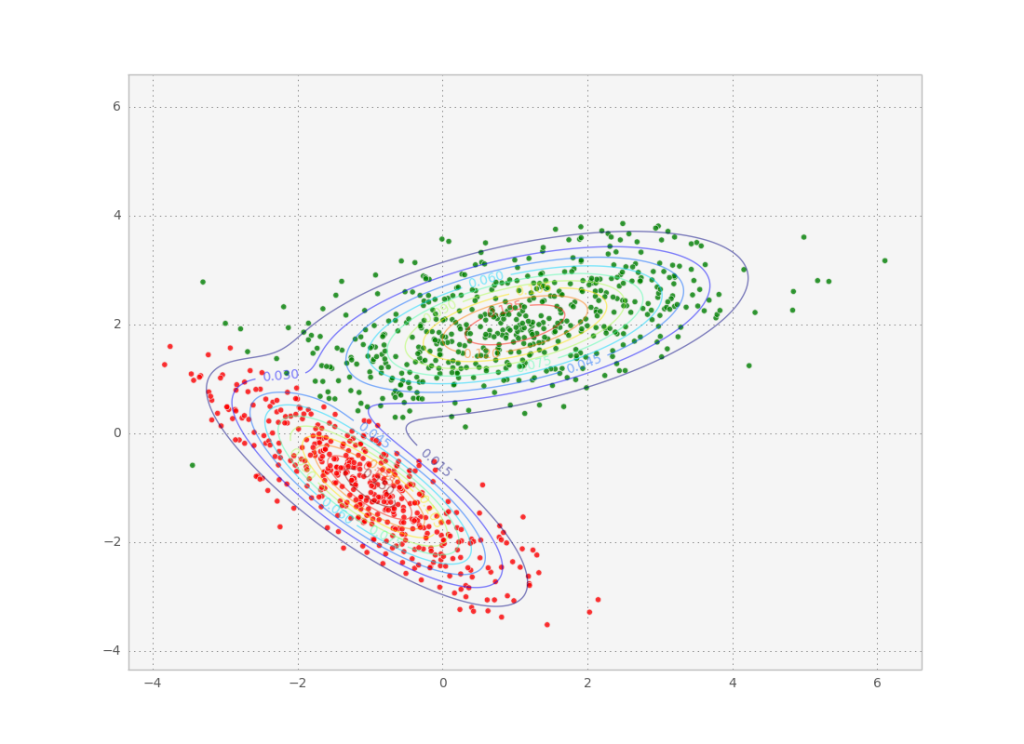One difficulty when using real data is that we don’t know how many components to use for the GMM, so we run the risk of over or underfitting the data. You might need to use another optimization loop on the number of components, using the BIC or the AIC as a cost function.

Below is the full code used to produce the images in this post.

import numpy as np
import matplotlib.pyplot as plt
import matplotlib.mlab as mlab
from mpl_toolkits.mplot3d import Axes3D
from sklearn import mixture

def q(x, y):
g1 = mlab.bivariate_normal(x, y, 1.0, 1.0, -1, -1, -0.8)
g2 = mlab.bivariate_normal(x, y, 1.5, 0.8, 1, 2, 0.6)
return 0.6*g1+28.4*g2/(0.6+28.4)

def plot_q():
fig = plt.figure()
ax = fig.gca(projection='3d')
X = np.arange(-5, 5, 0.1)
Y = np.arange(-5, 5, 0.1)
X, Y = np.meshgrid(X, Y)
Z = q(X, Y)
surf = ax.plot_surface(X, Y, Z, rstride=1, cstride=1, cmap=plt.get_cmap('coolwarm'),
linewidth=0, antialiased=True)
fig.colorbar(surf, shrink=0.5, aspect=5)

plt.savefig('3dgauss.png')
plt.clf()

def sample():
'''Metropolis Hastings'''
N = 10000
s = 10
r = np.zeros(2)
p = q(r, r)
print p
samples = []
for i in xrange(N):
rn = r + np.random.normal(size=2)
pn = q(rn, rn)
if pn &gt;= p:
p = pn
r = rn
else:
u = np.random.rand()
if u &lt; pn/p:
p = pn
r = rn
if i % s == 0:
samples.append(r)

samples = np.array(samples)
plt.scatter(samples[:, 0], samples[:, 1], alpha=0.5, s=1)

'''Plot target'''
dx = 0.01
x = np.arange(np.min(samples), np.max(samples), dx)
y = np.arange(np.min(samples), np.max(samples), dx)
X, Y = np.meshgrid(x, y)
Z = q(X, Y)
CS = plt.contour(X, Y, Z, 10, alpha=0.5)
plt.clabel(CS, inline=1, fontsize=10)
plt.savefig("samples.png")
return samples

def fit_samples(samples):
gmix = mixture.GMM(n_components=2, covariance_type='full')
gmix.fit(samples)
print gmix.means_
colors = ['r' if i==0 else 'g' for i in gmix.predict(samples)]
ax = plt.gca()
ax.scatter(samples[:,0], samples[:,1], c=colors, alpha=0.8)
plt.savefig("class.png")

if __name__ == '__main__':
plot_q()
s = sample()
fit_samples(s)


# An introduction to the metropolis method with python

I already talked about MCMC methods before, but today I want to cover one of the most well known methods of all, Metropolis-Hastings. The goal is to obtain samples according to to the equilibrium distribution of a given physical system, the Boltzmann distribution. Incidentally, we can also rewrite arbitrary probability distributions in this form, which is what allows for the cross pollination of methods between probabilistic inference and statistical mechanics (look at my older post on this). Since we don’t know how to sample directly from the boltzmann distribution in general, we need to use some sampling method.

The easiest would be to do rejection sampling. Draw a random number x uniformly and reject it with probability P(x). For high dimensional systems, this would require an astronomical number of samples, so we need to make sure we draw samples likely to get accepted. To do so, we draw the samples from a markov chain: a stochastic process which moves from one state of the system (i.e. a given configuration of variables) to another arbitrary state with some probability. Intuitively, if we set up the markov chain so that it moves preferentially to states close to the one we were in, they are more likely to get accepted. On the other hand, the samples will be correlated, which means we cannot draw all samples generated by the chain, but need to wait some time after drawing a new independent sample (given by the autocorrelation of the chain).

The markov chain needs to obey certain principles: ergodicity, which means the chain is able to reach all states in the system from any initial state (to guarantee the probability distribution is represented correctly); and detailed balance, which means the probability of going from state A to state B is the same as that of from state B to A, for all pairs of states in the system. This makes sure the chain doesn’t get stuck in a loop where it goes from A to B to C and back to A. Mathematically, the probabilities must obey

$$\frac{p_{A\rightarrow B}}{p_{B\rightarrow A}}=e^{-\beta (E_B - E_A)}$$

for the boltzmann distribution.

Now, any markov chain with those properties will converge to the required distribution, but we still haven’t decided on a concrete transition rule $p_{A\rightarrow B}$. The clever part about metropolis hastings comes now. Once a new state B has been proposed, we can actually choose to not transition to it, and instead stay where we are without violating detailed balance. To do so, we define the acceptance ratio A and the proposal distribution g. The two of them must be balanced such that

$$\frac{p_{A\rightarrow B}}{p_{B\rightarrow A}}=\frac{g_{A\rightarrow B}A_{A\rightarrow B}}{g_{B\rightarrow A}A_{B\rightarrow A}}$$

We can choose a symmetric g for simplicity, such that $g_{A\rightarrow B}=g_{B\rightarrow A}$, and thus g cancels out. Then

$$\frac{A_{A\rightarrow B}}{A_{B\rightarrow A}}=e^{-\beta (E_B - E_A)}$$

Now what we want is to accept as many moves as possible, so we can set one of the A’s to 1 and the other to the value on the right hand side of the equation. Because the most likely states of the system are the ones with low energy, we generally want to move in that direction. Thus we choose the A’s to follow the rule

$$A_{A \rightarrow B} = \begin{cases}e^{-\beta (E_B - E_A)} & \text{if} \; E_B - E_A > 0 \\ 1 & \text{otherwise} \end{cases}$$

Again, this rule actually applies to any probability distribution, since you can go back and forth from the boltzmann form to an arbitrary distribution. Let’s look at how to implement this for a simple gaussian mixture. (The code is not very well optimized, but it follows the text exactly, for ease of understanding)

import numpy as np
import matplotlib.pyplot as plt
import matplotlib.mlab as mlab

def q(x, y):
g1 = mlab.bivariate_normal(x, y, 1.0, 1.0, -1, -1, -0.8)
g2 = mlab.bivariate_normal(x, y, 1.5, 0.8, 1, 2, 0.6)
return 0.6*g1+28.4*g2/(0.6+28.4)

'''Metropolis Hastings'''
N = 100000
s = 10
r = np.zeros(2)
p = q(r, r)
print p
samples = []
for i in xrange(N):
rn = r + np.random.normal(size=2)
pn = q(rn, rn)
if pn &gt;= p:
p = pn
r = rn
else:
u = np.random.rand()
if u &lt; pn/p:
p = pn
r = rn
if i % s == 0:
samples.append(r)

samples = np.array(samples)
plt.scatter(samples[:, 0], samples[:, 1], alpha=0.5, s=1)

'''Plot target'''
dx = 0.01
x = np.arange(np.min(samples), np.max(samples), dx)
y = np.arange(np.min(samples), np.max(samples), dx)
X, Y = np.meshgrid(x, y)
Z = q(X, Y)
CS = plt.contour(X, Y, Z, 10)
plt.clabel(CS, inline=1, fontsize=10)
plt.show()Result of the above code. As we wanted, the individual samples follow the desired probability distribution.

I guesstimated a reasonable value for the sampling rate (1 sample every 10 steps), but you could more rigorously calculate the autocorrelation for the markov chain and fit it to an exponential to get a correlation time estimate which is be a more appropriate guess. To do that, you need to calculate the autocorrelation of some observable of the probability distribution (i.e. the energy E). In this gaussian case, the autocorrelation time is so small I couldn’t even fit an exponential to the autocorrelation time the observables I tried.

# Pinch to zoom in libgdx

So I was a bit confused how to reproduce the multitouch gesture you often see in mobile gallery apps using libgdx. The idea is to zoom and recenter the viewport such that the points where your fingers are anchored are always the same (in game coordinates). Assuming you don’t need to rotate, here is the code I came up with:

public class MyGestures implements GestureListener {

/* more stuff....
*/
@Override
public boolean pinch(Vector2 initialPointer1, Vector2 initialPointer2,
Vector2 pointer1, Vector2 pointer2) {
//grab all the positions
touchPos.set(initialPointer1.x, initialPointer1.y, 0);
camera.unproject(touchPos);
float x1n = touchPos.x;
float y1n = touchPos.y;
touchPos.set(initialPointer2.x, initialPointer2.y, 0);
camera.unproject(touchPos);
float x2n = touchPos.x;
float y2n = touchPos.y;
touchPos.set(pointer1.x, pointer1.y, 0);
camera.unproject(touchPos);
float x1p = touchPos.x;
float y1p = touchPos.y;
touchPos.set(pointer2.x, pointer2.y, 0);
camera.unproject(touchPos);
float x2p = touchPos.x;
float y2p = touchPos.y;

float dx1 = x1n - x2n;
float dy1 = y1n - y2n;
float initialDistance = (float) Math.sqrt(dx1*dx1+dy1*dy1);
float dx2 = x1p - x2p;
float dy2 = y1p - y2p;
float distance = (float) Math.sqrt(dx2*dx2+dy2*dy2);

if(zooming == false) {
zooming = true;
cx = (_x1 + _x2)/2;
cy = (_y1 + _y2)/2;
px = camera.position.x;
py = camera.position.y;
initZoom = camera.zoom;
} else {
float nextZoom = (initialDistance/distance)*scale;
/* do some ifs here to check if nextZoom is too zoomed in or out*/
camera.zoom = nextZoom;
camera.update();

Vector3 pos = new Vector3((pointer1.x + pointer2.x)/2, (pointer1.y + pointer2.y)/2, 0f);
camera.unproject(pos);
dx = cx - pos.x;
dy = cy - pos.y;
/* do some ifs here to check if we are in bounds*/
camera.translate(dx, dy);
camera.update();
}
return false;
}
}


Of course, you shouldn’t put all this stuff into this method: each logical piece of code should be in its own method (and in minesweeper most of it is actually on another object, since I like to have only code relating to gesture handling on the gesture handler object)

# Simple pattern formation with cellular automata

A cellular automaton is a dynamical system where space, time and dynamic variable are all discrete. The system is thus composed of a lattice of cells (discrete space), each described by a state (discrete dynamic variable) which evolve into the next time step (discrete time) according to a dynamic rule.

$$x_i^{t+1} = f(x_i^t, \Omega_i^t, \xi)$$

This rule generally depends on the state of the target cell $x_i^t$, the state of its neighbors $\Omega_i^t$, and a number of auxiliary external variables $\xi$. Since all these inputs are discrete, we can enumerate them and then define the dynamic rule by a transition table. The transition table maps each possible input to the next state for the cell. As an example consider the elementary 1D cellular automaton. In this case the neighborhood consists of only the 2 nearest neighbors $\Omega_i^t = {x_{i-1}^t, x_{i+1}^t}$ and no external variables.

In general, there are two types of neighborhoods, commonly classified as Moore or Von Neumann. A Moore neighborhood of radius $r$ corresponds to all cells within a hypercube of size $r$ centered at the current cell. In 2D we can write it as $\Omega_{ij}^t = {x^t_{kl}:|i-k|\leq r \wedge |j-l|\leq r}\setminus x^t_{ij}$. The Von Neumann neighborhood is more restrictive: only cells within a manhattan distance of $r$ belong to the neighborhood. In 2D we write $\Omega_{ij}^t = {x^t_{kl}:|i-l|+|j-k| \leq r}\setminus x^t_{ij}$.

Finally it is worth elucidating the concept of totalistic automata. In high dimensional spaces, the number of possible configurations of the neighborhood $\Omega$ can be quite large. As a simplification, we may consider instead as an input to the transition table the sum of all neighbors in a specific state $N_k = \sum_{x \in \Omega}\delta(x = k)$. If there are only 2 states, we need only consider $N_1$, since $N_0 = r - N_1$. For an arbitrary number $m$ of states, we will obviously need to consider $m-1$ such inputs to fully characterize the neighborhood. Even then, each input $N_k$ can take $r+1$ different values, which might be too much. In such cases we may consider only the case when $N_k$ is above some threshold. Then we can define as an input the boolean variable

$$P_{k,T}=\begin{cases} 1& \text{if}\; N_k \geq T,\\ 0& \text{if}\; N_k < T. \end{cases}$$

In the simulation you can find here, I considered a cellular automaton with the following properties: number of states $m=2$; moore neighborhood with radius $r=1$; lattice size $L_x \times L_y$; and 3 inputs for the transition table:

• Current state $x_{ij}^t$
• Neighborhood state $P_{1,T}$ with $T$ unspecified
• One external input $\xi$
$$\xi_{ij}=\begin{cases} 1& \text{if}\; i \geq L_x/2,\\ 0& \text{if}\; i < L_x/2. \end{cases}$$
• Initial condition $x_{ij} = 0 \; \forall_{ij}$

For these conditions a deterministic simulation of these conditions yields only a few steady states: homogeneous 1 or 0, half the lattice 1 and the other 0, and oscillation between a combination of the previous.

One possibility would be to add noise to the cellular automaton in order to provide more interesting dynamics. There are two ways to add noise to a cellular automaton:

The most straightforward way is to perform the following procedure at each time step:

• Apply the deterministic dynamics to the whole lattice
• For each lattice site $ij$, invert the state $x_{ij}$ with probability $p$

This procedure only works of course for $m=2$. In the case of more states there is no obvious way to generalize the procedure and we need to use a proper monte carlo method to get the dynamics.

A second way is to implement a probabilistic cellular automaton. In this case the transition table is generalized to a markov matrix: each input is now mapped not to a specific state but rather to a set of probabilities for a transition to each state ($m$ probabilities). Naturally for each input these sum to one. In this case we have $m$ times more parameters than before.